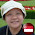## Wednesday, 17 February 2016

### Algebra... Different "Faces" of Algebra (II)

The perimeter of a rectangle is (6x + 5y) cm.
Suggest 2 possible dimensions of the rectangle.

e.g.
Length of rectangle = 2x + 2y cm
Breadth of rectangle = x + 0.5y cm
Check:
Perimeter = 2 (2x + 2y) + 2 (x + 0.5y) cm
= 4x + 4y + 2x + y cm
= 6x + 5y cm

Indicate any assumptions made.

1.Length: 2.5x + 1.5y
Breath: 0.5x + 1y
Check: 2(2.5x + 1.5y) + 2(0.5x + 1y)
=6x + 5y cm
____________________________________________
Length: 2.75x + 2y cm
Breath: 0.5x + 0.5y cm
Check: (2(2.75x + 2y) + 2(0.5x + 0.5y))
=6x + 5y cm

2.Length: 3x

1.2nd)
Length: 2.5y

3.Length=(2.1y+3x)
Perimeter=2(2.5y+3x)cm=(5y+6x)cm

4.1>
Length: 3x
Breath: 2.5y
Perimeter: 2(3x)+2(2.5y)=(6x+5y)cm

2>
Length: 2.5y
Breath: 3x
Perimeter: 2(2.5y)+2(3x)=(6x+5y)cm

5.Length:(-2x+5x)cm
Perimeter:2(-2x+5x)+(-4y+5y+1.5y)=(6x+5y)cm

6.1) Length: (2x+1.5y)cm
Check: (4x +3y)cm + ( 2x+2y)cm = (6x+5y) cm
2) Length: ( 1.5x+2y)cm
Check: (3x+4y) + (3x+1y) cm = 6x+5y

7.1)Length:2x-0.5y
Check:(2x+x+3y-0.5y)X2=(3x+2.5y)X2
=6x+5y
2)Length:4x-y
Check:(4x-x)+(3.5y-y)=3x+2.5y
(3x+2.5)yX2=6x+5y

8.Length:3x-1
Check:(3x-1)+(2.5y+1)+(3x-1)+(2..5y+1)=(6x)-2+(5y)+2=6x+5y

9.Length: (2x + 2.5y)cm
Breath: x cm
Check:
Perimeter=(2x + 2.5y) cm x2 + x cm x2=(6x + 5y)cm

10.1.How do you find the perimeter of a rectangle?
What's the formula?

11.Length: (3X + 1.5Y)cm